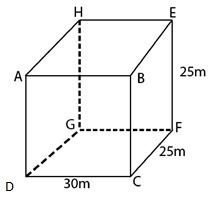Guru

# A small indoor greenhouse (herbarium) is made entirely of glass panes (including base) held together with tape. It is 30cm long, 25 cm wide and 25 cm high. (i)What is the area of the glass? (ii)How much of tape is needed for all the 12 edges? Q.6

• -1

sir what is the best way for solving the question of class 9th chapter of Surface areas and Volumes chapter of question no.1 of math of exercise 13.1    of math, what is the best way for solving the question of class 9th ncert book of question no. 6 A small indoor greenhouse (herbarium) is made entirely of glass panes (including base) held together with tape. It is 30cm long, 25 cm wide and 25 cm high. (i)What is the area of the glass? (ii)How much of tape is needed for all the 12 edges?

Share

1. Length of greenhouse, say l = 30cm

Breadth of greenhouse, say b = 25 cm

Height of greenhouse, say h = 25 cm

(i) Total surface area of greenhouse = Area of the glass = 2[lb+lh+bh]

= [2(30×25+30×25+25×25)]

= [2(750+750+625)]

= (2×2125) = 4250

Total surface area of the glass is 4250 cm2

(ii)From figure, tape is required along sides AB, BC, CD, DA, EF, FG, GH, HE AH, BE, DG, and CF.

Total length of tape = 4(l+b+h)

= [4(30+25+25)] (after substituting the values)

= 320

Therefore, 320 cm tape is required for all the 12 edges.

• 0